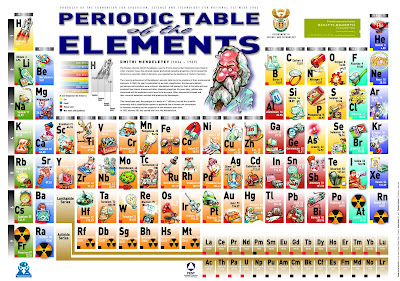## Saturday, May 2, 2009

### Chemistry - The Mole

The Chemistry question is,
An organic compound contains 12.8% carbon, 2.1% hydrogen and 85.1% bromine by mass. Its relative molecular mass is 188.
(a) Calculate the empirical formula
(b) Deduce its molecular formulaExtracted from
Solution:
The percentage of each element is directly proportional to its mass in grams. That is, the mass of each element in 100g of the compound is equal to its percentage in the compound.

The relative atomic mass of each element can be found from the periodic table.Extracted from http://aleptu.com/images/2009/03/periodic_table_of_pokemon.jpg
Humorous Periodic Table with Pokemons for the elements - very educational and creative. Very rare and precious treats for all Pokemon lovers.Extracted from http://www.lbl.gov/abc/marsh-nuclei/images/table_sig.jpg
Serious Periodic Table with atomic mass, states and metal/non-metal/gas/others properties for the elements - very educational and professional.

Knowing the mass and relative atomic mass of each element, the number of moles of the element can be calculated by using the formula:

Number of moles of an elementFrom the question above, the Mass of carbon is 12.8g (in 100g of the compound)
From the periodic table,
Relative atomic mass of carbon, Ar, is 12.

Therefore the Number of moles of carbon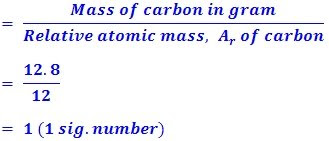The number of moles of the hydrogen and bromine can be calculated using the same method.

The Molar ratio is also calculated by taking the number of moles of each element and divide by the smallest mole number. In this case the smallest mole number is 1.

The results are tabulated as follow.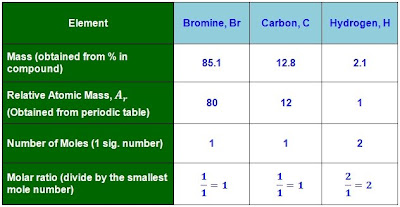Finding the number of moles of bromine, carbon and hydrogen in the compound

(a) The empirical formula

The empirical formula of a compound shows
i) The types of elements present in it,
ii) The simplest ratio of the different types of atoms in it.

The empirical formula of the compound is thus,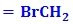(b) The molecular formula

The molecular formula is the formula that shows the exact number of atoms of each element in a molecule.
The molecular formula of a compound is a multiple of its empirical formula.

If the empirical formulathen the molecular formula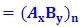n can be found using the equation: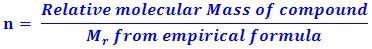Given that the relative molecular mass of the compound is 188

Therefore, n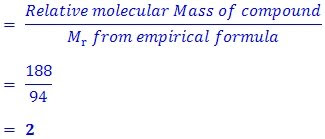The empirical formula of the compound isThen the molecular formula